# Integral of sin(x)/x from 0 to infinity | A satisfying solution

Today we have a tough integral: not only is this a special integral (the sine integral Si(x)), but it also goes from 0 to infinity! Because of the first characteristic, there is no elementary antiderivative and therefore we can’t simply plug the bounds into the result; this means none of the techniques we know of will work. We need to use a different approach: differentiation under the integral sign (also known as Feynman technique).

The idea is that we introduce a parameter so that, when differentiating (with respect to the parameter) a certain function that contains it, something happens that will make the integral easier to evaluate. In this case, in order to make this integral easy, we need to get rid of that x at the denomitator; to do so we need to differentiate a function that contains a parameter multiplied by x so that the x is generated and the one at the denominator will cancel out with it.

### Generalized form of the integral of sin(x)/x

You may think we can do something like this: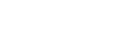we take the derivative with respect to a and we get xcos(ax)/x=cos(ax); okay, this is an easy integral… well, no, and this is why:

The integral goes from 0 to infinity, and at x=infinity, sin(ax) and cos(ax) are not defined. This means this is not the right way to go. What can we do then?

We need to multiply the integrand by another function so that the x can be generated while keeping the integral convergent. I am talking about the exponential function!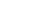At x=0 the output is 1 and at x=infinity the result is 0, therefore this function converges. Also, if we take the derivative with respect to alpha, the x will be generated!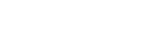This is the integral we are going to work with, and since it is in terms of a parameter, we will write it like this: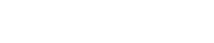This means that the integral we had at the beginning is I(0), since e^(0)=1: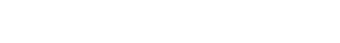As I said before, we want to take the derivative with respect to the parameter, so: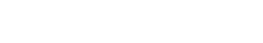or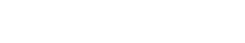An integral is a sum, so the total derivative of the whole sum is the partial derivative of every addend. The symbol is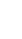So the derivative of an integral is the partial derivative of the integrand, therefore:I don’t know you, but I think this looks amazing! Anyway, let’s take the derivative: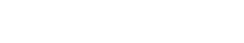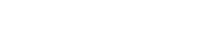This is so much better! In my previous post we found the answer to this integral: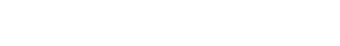and so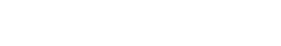At this point we can plug in the bounds: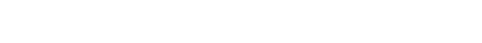The first term is equal to 0 because e to the –infinity equals 0 and 0 multiplied by any number gives 0; this means we are left with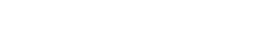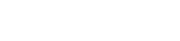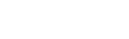Great! Now, in order to find I(a), we need to take the antiderivative on both sides: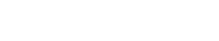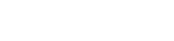If you remember, this is one of the fundamental integrals, and the result is arctan(a);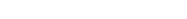The integral we had at the beginning is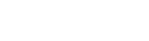so you might think about doing the following: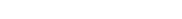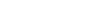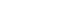This is pointless; we need to find the constant C by plugging in some other number. Look what happens with I(infinity):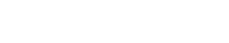This is equal to 0, since e^(-infinity) = 0 and so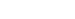Also,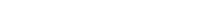therefore,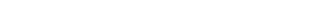Although this might look crazy, the answer is really nice! The tangent of what angle in radiants is equal to infinity? pi/2!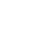Now that we have the constant, we can write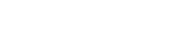At this point we can plug in 0 in order to have the starting integral and its corresponding answer: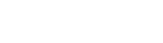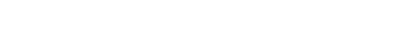Hence,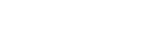To recap:

###### Differentiation under the integral sign
• find a function of a parameter
• differentiate with respect to that parameter
• solve the new integral
• plug in a number that cancels the integral to find the constant C
• plug in the original value of the parameter

If you liked this post give it a like! If you have any doubts, questions, suggestions, leave a comment and I’ll be happy to reply! Subscribe for more!

Rating: 5 out of 5.

Join 31 other subscribers.

{{#message}}{{{message}}}{{/message}}{{^message}}It appears your submission was successful. Even though the server responded OK, it is possible the submission was not processed. Please contact the developer of this form processor to improve this message. Learn more{{/message}}

Submitting…